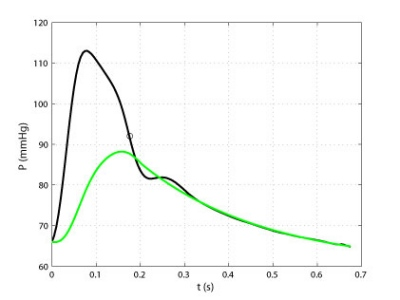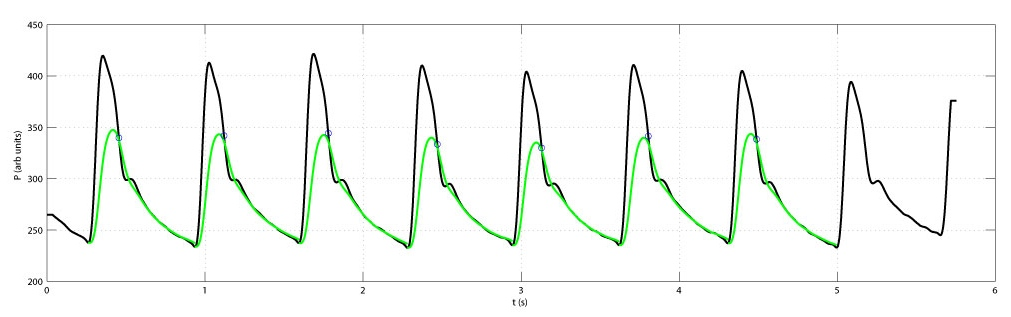# the calculation of reservoir pressure

It is probably obvious to anyone reading these pages that we have defined the reservoir pressure and shown some examples, but still have not explained how to calculate it. We have developed an algorithm for estimating the reservoir pressure from the measured arterial pressure and will now describe how it is done.

First we observe that it is practically impossible to solve for the reservoir pressure using the time-delay ODE derived from the conservation of mass. The solution depends on knowing the compliances and resistances for every artery; information that is not available clinically (or even experimentally). We therefore must rely on approximate methods.

All of the methods of estimating the reservoir pressure are based on the assumption that the wave delay times are small, τn << 1. This can be justified by looking at the measured wave transit times where the time for the initial compression wave to traverse the whole of the aorta is less than 50 ms while the time from minimum to maximum pressure is of the order of 200 ms. Thus, expanding Pn(t - τn) in a Taylor expansion, we obtain

Pres(t-τn) = Pres(t) + O(τn)

where O(τn) stands for terms of order τn. When we substitute this into the mass conservation equation, the terms involving Pres can be taken out of the summations and we obtain the ordinary differential equation

C dPres/dt = Q0 - (Pres - P)/R

where, as in the derivation of the Windkessel pressure, C = ΣN Cn and 1/R = ΣK 1/Rk. This shows that to O(τn), the equation for Pres is the same as the equation for PWk. This is the basis of our approximate calculation of Pres.

### Pressure and aortic flow known

If the aortic inflow Q0(t) is measured simultaneously with the pressure P0(t), then the calculation of Pres(t) is relatively straightforward. The solution of the above ODE is easily found by quadrature

(Pres-P) = exp(-t/RC)/C ∫0t Q0(s) exp(s/RC) ds + (Pres(0)-P) exp(-t/RC)

Note that during diastole when the valve is closed, Q0 = 0 and the solution for Pres is and exponentially falling function of time with the time constant RC. This provides a convenient way to determine the arterial properties R and C. If we fit a falling exponential function to the measured pressure during diastole, we can obtain the time constant RC and the asymptote P. We can then find the net arterial resistance from the mean of the measured pressure and flow rate, R = <P>/<Q>. Knowing R we can obtain C using the time constant RC.

We use this method of calculating Pres in experiments where we measure both the pressure and the flow rate in the ascending aorta. Clinically, however, it is difficult to obtain simultaneous measurement of pressure and flow and so we have developed another more approximate method for calculating Pres when only the pressure is measured.

### Only pressure known

The method for calculating Pres from a pressure measurement only is based on the observation in our experiments that the excess pressure P-Pres is proportional to the flow in the aortic root Q0. If we assume that this is true, we can substitute Q0 = aC(P-Pres into the mass conservation equation, where a is an unknown constant of proportionality and we have included C for algebraic simplicity. If we also write b=1/RC for convenience, the equation takes the form

d(Pres-P)dt + (a+b)(Pres-P) = a(P(t)-P)

This equation is similar in form to the previous ODE, but the right hand side depends on P(t) rather than Q0. The solution is so similar that we do not write it out here. The same procedure is followed to determine RC and P and all that remains is to determine the unknown constant a. This is done by iteratively finding the value of a that minimises the square difference between the calculated Pres during diastole. An algorithm for carrying out this calculation has been developed and we plan to make it public on these pages together with a detailed description of the method.

All of the examples of Pres in these pages have been calculated using the reservoir pressure algorithm described briefly above. It has been extensively tested for experimental data where the aortic flow is known and shown to be satisfactory. It has also been tested extensively on clinical data where the aortic flow is not known and so the 'right' answer cannot be determined. However, it does give reasonable answers for the vast majority of cases and has proven to be very robust. For example, when applied to the Cafe data base of more than 2032 pressure waveforms it failed to converge in only 9 cases.

Results for one of the radial pressure waveforms are shown in the following figures:This figure shows the pressure measured in the radial artery using applanar tonometry (black) and the reservoir pressure calculated using the reservoir pressure algorithm (green). The circle represents the time at which dP/dt is minimum which we take as the start of diastole in the algorithm. Most people take the minimum of the dicrotic notch as the start of diastole, but there is some evidence that the (dP/dt)min point correlates better with the time of closure of the aortic valve when pressure measurements are made in the root of the aorta. This point is more convenient in measurements made at other locations because it always exists whereas the dicrotic notch tends to disappear as the pressure wave moves distally.This figure shows the raw data that was used to calculate the ensemble average pressure waveform shown in the figure above. The reservoir pressure algorithm can be used beat by beat to find calculate the reservoir pressure for each individual beat. The measured pressure is in black, the reservoir pressure is in green. The circle again identifies the time of dP/dt)min.

A pdf file summarising the mathematical details of the definition of the reservoir/excess pressure and the demonstration that the reservoir pressure represents the minimum hydraulic work that the ventricle can do to generate a given flow for the given arterial resistance and compliance is here: